### Home > CALC > Chapter 9 > Lesson 9.2.1 > Problem9-53

9-53.
1. The graph below shows f". Use it to answer the following questions. Homework Help ✎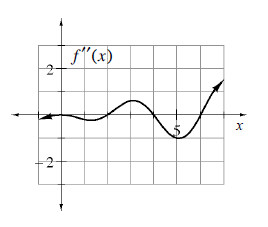1. Over what interval(s) is f concave up?

2. Estimate f '''(5).

3. At what x-value(s) does f have a point of inflection?

4. At what x-value(s) does f ' have a minimum value?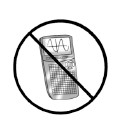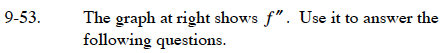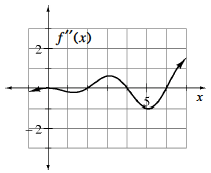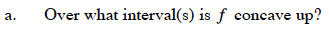f is concave up when f ″ is positive. Where is the given graph positive?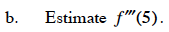This is asking for the slope of the given graph at x = 5.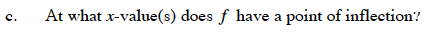This is where f ″ changes sign. Where does the given graph change from negative to positive and vice versa?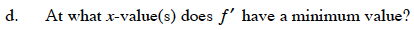f ′ has a minimum where its slope changes from negative to positive.
The slope of f ′ is f ″. So where does f ″ change from negative to positive?